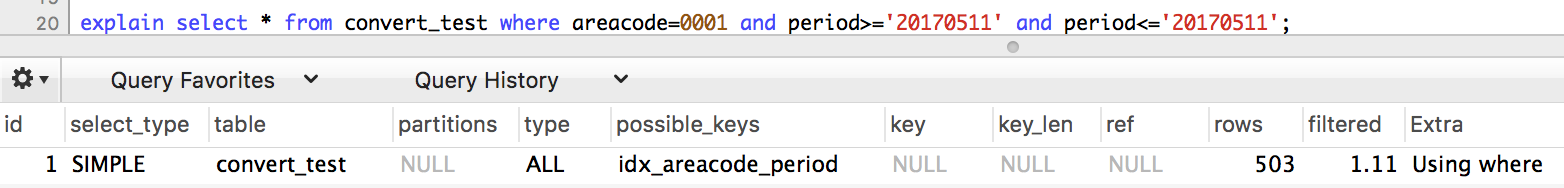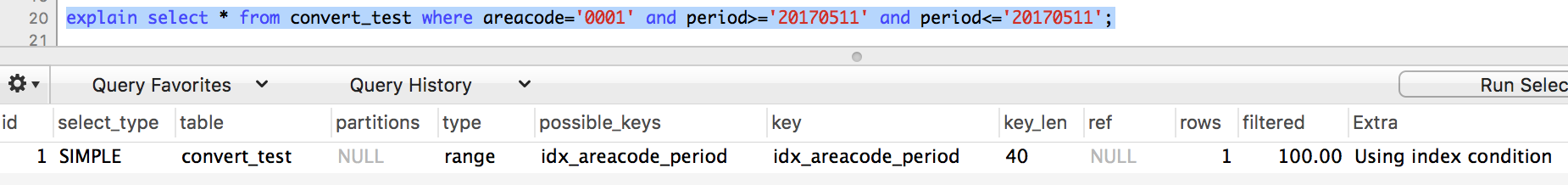### 现象

``````select * from convert_test where areacode=0001 and period>='20170511' and period<='20170511';
``````

`convert_test`表结构如下：

``````CREATE TABLE `convert_test` (
`id` bigint(20) unsigned NOT NULL AUTO_INCREMENT,
`areacode` char(12) NOT NULL DEFAULT '',
`period` int(6) unsigned NOT NULL DEFAULT 0,
`mid_price` int(10) unsigned NOT NULL DEFAULT 0,
`mid_change` float NOT NULL DEFAULT 0,
`updated_datetime` timestamp NOT NULL DEFAULT CURRENT_TIMESTAMP ON UPDATE CURRENT_TIMESTAMP,
PRIMARY KEY (`id`),
UNIQUE KEY `idx_areacode_period` (`areacode`,`period`)
) ENGINE=InnoDB DEFAULT CHARSET=utf8 COMMENT='隐式转换测试表';
``````

``````mysql> explain select * from convert_test where areacode=0001 and period>='20170511' and period<='20170511';
``````### 原因

``````mysql> explain select * from convert_test where areacode='0001' and period>='20170511' and period<='20170511';
``````### 扩展

1. 两个参数至少有一个是 NULL 时，比较的结果也是 NULL，例外是使用 <=> 对两个 NULL 做比较时会返回 1，这两种情况都不需要做类型转换
2. 两个参数都是字符串，会按照字符串来比较，不做类型转换
3. 两个参数都是整数，按照整数来比较，不做类型转换
4. 十六进制的值和非数字做比较时，会被当做二进制串
5. 有一个参数是 TIMESTAMP 或 DATETIME，并且另外一个参数是常量，常量会被转换为 timestamp
6. 有一个参数是 decimal 类型，如果另外一个参数是 decimal 或者整数，会将整数转换为 decimal 后进行比较，如果另外一个参数是浮点数，则会把 decimal 转换为浮点数进行比较
7. 所有其他情况下，两个参数都会被转换为浮点数再进行比较

``````select * from convert_test where areacode=0001 and period>='20170511' and period<='20170511';
select * from convert_test where areacode=1 and period>='20170511' and period<='20170511';
select * from convert_test where areacode=0001.0 and period>='20170511' and period<='20170511';
select * from convert_test where areacode=1.0 and period>='20170511' and period<='20170511';
``````

mysql 在查询的时候，会将areacode转换成浮点型进行比较